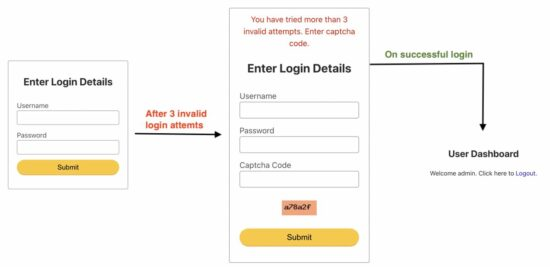In this tutorial, we will show the captcha code if a user has tried more than three failed login attempts. In the previous tutorial, we have seen the user login and PHP captcha. So, we will combine these two tutorials with adding a captcha control for invalid login.

In this example, we have a MySQL table to add failed login entries. We calculate the number of failed attempts based on the client’s IP Address. If this count exceeds 3, the captcha code will be displayed to the user.

This code contains a login form with the captcha code. The captcha code will be displayed when the user tries over three invalid attempts.

``````<form name="frmUser" method="post" action="">
<div class="validation-message"><?php if (\$message != "") {
echo \$message;
} ?></div>
<div class="row">
<label class="text-left">Username</label> <input type="text" name="user_name" class="full-width">
</div>
<div class="row">
</div>
<div class="row">
</div>
<div class="m-20">
</div>
<?php } ?>
<div class="row">
<input type="submit" name="submit" value="Submit" class="full-width">
</div>
</form>
``````

## PHP Code for Calculating Failed Login Count

This code calculates the number of invalid login attempts based on the IP address.

``````<?php
\$conn = mysqli_connect('localhost', 'root', '', 'db_login');
\$sql = "SELECT count(ip_address) AS failed_login_attempt FROM failed_login WHERE ip_address = ? AND date BETWEEN DATE_SUB( NOW() , INTERVAL 1 DAY ) AND NOW()";
\$stmt = \$conn->prepare(\$sql);
\$stmt->bind_param('s', \$ip);
\$stmt->execute();
\$result = \$stmt->get_result();
\$row = \$result->fetch_assoc();
\$result->free();
?>
``````This code validates user credentials and if the credentials are invalid, then an entry with ip_address and date will be added to the database.

``````<?php
session_start();
\$message = "";
\$message = "Enter Correct Captcha Code";
}
\$conn = mysqli_connect('localhost', 'root', '', 'db_login');
\$sql = "SELECT count(ip_address) AS failed_login_attempt FROM failed_login WHERE ip_address = ? AND date BETWEEN DATE_SUB( NOW() , INTERVAL 1 DAY ) AND NOW()";
\$stmt = \$conn->prepare(\$sql);
\$stmt->bind_param('s', \$ip);
\$stmt->execute();
\$result = \$stmt->get_result();
\$row = \$result->fetch_assoc();
\$result->free();
if (count(\$_POST) > 0 && \$captcha == true) {
\$query = "SELECT * FROM users WHERE user_name=?";
\$stmt = \$conn->prepare(\$query);
\$stmt->bind_param('s', \$_POST["user_name"]);
\$stmt->execute();
\$result = \$stmt->get_result();
\$row = \$result->fetch_assoc();
\$result->free();
\$isSuccess = 0;
if (is_array(\$row)) {

\$isSuccess = 1;
}

if (\$isSuccess == 1) {
\$_SESSION["user_id"] = \$row["id"];
\$_SESSION["user_name"] = \$row["user_name"];
\$stmt = \$conn->prepare(\$sql);
\$stmt->bind_param('s', \$ip);
\$stmt->execute();
}
}
else {
\$stmt = \$conn->prepare(\$sql);
\$stmt->bind_param('s', \$ip);
\$stmt->execute();
} else {
\$message = "You have tried more than 3 invalid attempts. Enter captcha code.";
}
}
}
?>
``````

## Allow user to access the application dashboard on successful login

After validating the entered login, if everything goes well, then the user login session will be created. In this successful case, the PHP session is set with a user-defined index to store the logged-in state.

This code sets the session with an index user_id to store the logged-in user id. The below PHP code checks if the session is not empty. If so, it will allow the user to see the application dashboard.

``````<?php
if (isset(\$_SESSION["user_id"])) {
}
?>
``````

And the dashboard page code is below. It will show a welcome message to the logged-in user with a logout option.

``````<?php
session_start();
?>
<html>

<body>
<h2 class=" phppot-container text-center">User Dashboard</h2>
<div class="text-center">
<?php
if (\$_SESSION["user_name"]) {
?>